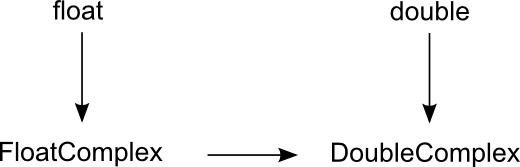# NMath User's Guide

3.1 Creating Complex Numbers (.NET, C#, CSharp, VB, Visual Basic, F#)

This section describes how to construct instances of FloatComplex and DoubleComplex.

Creating Complex Numbers from Numeric Values

You can construct complex number objects from a pair of numeric values representing the real and imaginary parts. If only a single value is passed, it is assumed to be the real part, and the imaginary part is set to 0.0. For example:

Code Example – C# complex numbers

```var c = new FloatComplex( 1.3, 4.5 );   // 1.3 + 4.5i
```
```var c2 = new DoubleComplex( 6.5 );       // 6.5 + 0.0i
```

Code Example – VB complex numbers

```Dim C As New FloatComplex(1.3, 4.5)   ' 1.3 + 4.5i
```
```Dim C2 As New DoubleComplex(6.5)      ' 6.5 + 0.0i
```

The static FromPolar() function constructs a complex number with a given magnitude and phase angle:

Code Example – C# complex numbers

```var c = DoubleComplex.FromPolar( 2 * Math.Sqrt(2), Math.PI/4 );
```
```// c = 2.0 + 2.0i
```

Code Example – VB complex numbers

```Dim C As DoubleComplex =
```
```  DoubleComplex.FromPolar(2 * Math.Sqrt(2), Math.PI / 4)
```
```' c = 2.0 + 2.0i
```

Creating Complex Numbers from Strings

You can also construct complex number types from a string representation of the form (real,imag). The parentheses are optional, and whitespace is ignored. Again, if only one value is supplied, it is assumed to be the real part. For instance, these are valid strings:

```4.2,-5.1
```
```(4.2,-5.1)
```
```4.2
```

These are not valid strings:

```4.2 - 5.1i
```
```4.2 - 5.1
```

Thus:

Code Example – C# complex numbers

```string s = "(1.1, -3.23)";
```
```var c = new DoubleComplex( s );
```

Code Example – VB complex numbers

```Dim S As String = "(1.1, -3.23)"
```
```Dim C As New DoubleComplex(S)
```

The static Parse() method performs the same function:

Code Example – C# complex numbers

```string s = "(1.1, -3.23)";
```
```DoubleComplex c = DoubleComplex.Parse( s );
```

Code Example – VB complex numbers

```Dim S As String = "(1.1, -3.23)"
```
```Dim C As DoubleComplex = DoubleComplex.Parse(s)
```

NOTE—Note that you cannot use parentheses to represent negative numbers, as is done in some financial formats, when parsing complex number strings.

Conversely, the overridden ToString() member function returns a string representation of complex number:

Code Example – C# complex numbers

```var c = new FloatComplex( 7.61, -1.2 );
```
```Console.WriteLine( c.ToString() );  // prints "(7.61,-1.2)"
```

Code Example – VB complex numbers

```Dim C As New FloatComplex(7.61, -1.2)
```
```Console.WriteLine(c.ToString())  ' prints "(7.61,-1.2)"
```

A variant of the ToString() method also accepts a standard .NET numeric format string. For example, the format string "E" indicates exponential (scientific) notion.

Implicit Conversion

The implicit conversion operators for the complex number classes are shown in Figure 1. An arrow indicates implicit promotion.

Figure 1 – Implicit conversion for complex numbersTop

Top